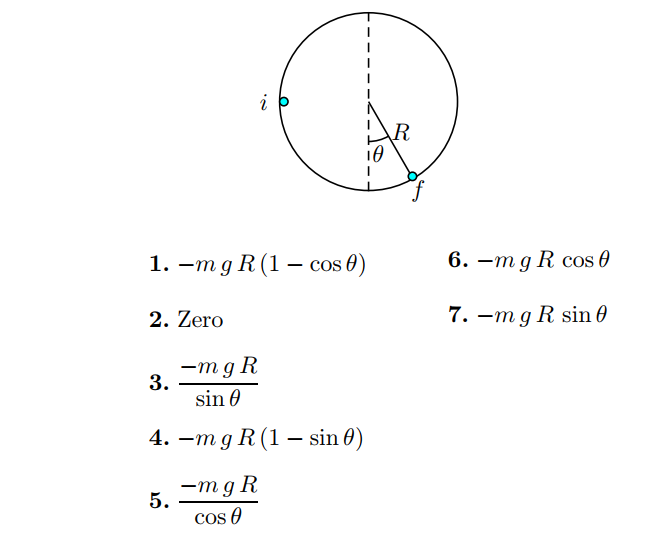Problem: A tiny object of mass m is sliding around inside a pipe of inner radius  R. When we first see the object it is momentarily at rest halfway up the side of the pipe. A moment later, it is momentarily at rest at an angle θ &lt; 90◦ from the vertical on the other side of the pipe. If a force of kinetic friction acted on the object as it slid, how much work did friction do in the time between the two instants of momentary rest?

FREE Expert Solution
79% (285 ratings)
Problem Details

A tiny object of mass m is sliding around inside a pipe of inner radius  R. When we first see the object it is momentarily at rest halfway up the side of the pipe. A moment later, it is momentarily at rest at an angle θ < 90 from the vertical on the other side of the pipe.

If a force of kinetic friction acted on the object as it slid, how much work did friction do in the time between the two instants of momentary rest?